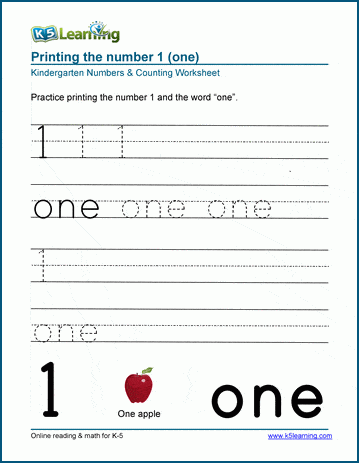# Math Worksheets by Topic

## Free math worksheets

Our math worksheets cover the full elementary school math curriculum.  All worksheets are printable pdf documents; answers are on the 2nd page.  No login or registration required.

Learning Numbers

Recognizing and printing numbers, ordinal numbers and odd / even numbers.

Counting

Counting objects, sequential counting, skip counting & counting backwards.

Comparing Numbers

Concepts of more, less, most, and least, comparing groups of objects, comparing numbers & ordering numbers.

Place Value

Base 10 blocks, tens and ones, composing and decomposing numbers, normal and expanded form, place values of up to 6 digit numbers.

Rounding

Rounding numbers of varying sizes to the nearest 10, 100 or other power of 10.

From counting a few objects using pictures up to adding multiple large numbers in columns with regrouping.

Subtraction

From subtracting by "taking away" objects using pictures to subtracting large numbers in columns with regrouping.

Multiplication

From basic multiplication facts to multiplying multi-digit whole numbers in columns.

Division

From basic division facts to long division of whole numbers with/without remainders.

Order of operations (PEMDAS)

Order of operations focused on the 4 operations and use of parenthesis.

Roman numerals

Fractions

From the concepts of "equal parts" through adding, subtracting, multiplying and dividing proper and improper fractions and mixed numbers.

Decimals

From converting simple fractions to/from decimals to the long division of multi-digit decimals.

Measurement

From size comparisons and measuring in non-standard units to length, weight, capacity and temperature in customary and metric units.

Money

Identifying coins and their values, counting money and shopping problems.

Time

The units of time, telling time, elapsed time, am/pm and calendars.

Geometry

Basic shapes, the classification and properties of 2D shapes, area and perimeters, classification of angles, plotting on coordinate grids and basic 3D shapes.

Factoring & prime factors

Factors, prime factors, greatest common factors (GCFs) and least common multiples (LCMs).

Exponents

Exponent notation including whole number, fractional and decimal bases, negative exponents, equations with exponents.

Proportions

Whole number and decimal proportions and proportion word problems.

Percents

Common calculations involving percents.

Integers

Absolute values, addition, subtraction, multiplication and division of integers (whole numbers which can be negative or positive).

Data & Graphing

Data collection, making and reading graphs and a little introductory probability and statistics.

Algebra

Introducing variables, constants, expressions and equations.

Word problems

Word problems covering most of the elementary school curriculum.Sample Math Worksheet

What is K5?

K5 Learning offers free worksheets, flashcards and inexpensive workbooks for kids in kindergarten to grade 5. Become a member to access additional content and skip ads.